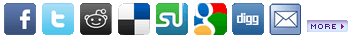Related Sites

 Related Books 1The Self-Driving Project: Using Artificial Intelligence to Deliver Project SuccessMS Project: Understanding How Microsoft Project Thinks Posted on Thursday, August 21 @ 07:47:34 EDT by webadminThere are a number of factors that dictate how Microsoft Project “thinks”.

The two main factors are:

• The Scheduling Formula
The Scheduling Formula leverages three variables:

• Units - The percentage of time required by a resource or resources to complete the task
• Duration - The number of working days required to complete the task
• Work - The effort required to complete the task
Many people say that the scheduling formula is: Units x Duration = Work

When you initially assign a Resource to a Task, this is the formula that is leveraged. Let’s demonstrate:

We will create Task 1 that is 5 days in Duration

We will now assign a Resource to this task

As soon as we assign this Resource to this task, Work changes to 40 hours.

Here is the how that number was calculated:

Units x Duration = Work
100% x (5*8) = 40
Or
100% x 40 = 40 hours

But using simple math, we can re-write our equation to solve for a different variable.

Our original formula solves for Work:
Units x Duration = Work

But we could re-write the formula to solve for Units:
Work / Duration = Units

Or we could re-write the formula to solve for Duration:
Work / Units = Duration

So we have shown that The Scheduling Formula can actually be written three different ways:

Units x Duration = Work
Work / Duration = Units
Work / Units = Duration

Now let’s prove that Microsoft Project also thinks this way.

In order to demonstrate this, we must introduce The Task Type or the Type field:

By default, Type is set to Fixed Units. But there are actually three different Task Type variables:

• Fixed Duration
• Fixed Units
• Fixed Work

Now to test Microsoft Project

Using our original scenario, let’s force Microsoft Project to solve for Duration.

We will leave Type set to Fixed Units and change Work to 80 hrs

Here is how Duration was calculated:

Work / Units = Duration
80 / 100% = 80
Or
80 / 100% = (80 / 8)
Or
80 / 100% = 10 days

Returning to our original scenario, let’s force Microsoft Project to solve for Work.

Leave Type set to Fixed Units and enter 10 days Duration

Here is how Work was calculated:

Units x Duration = Work
100% * 10 days = 80
Or
100% * 10 days = (10 * 8)
Or
100% * 10 days = 80 hours Work

Returning to our original scenario, let’s force Microsoft Project to solve for Units

Change Type to Fixed Duration and enter 20 hours Work

Here is how Units was calculated:

Work / Duration = Units
20 / 5 days = 50%
Or
20 / 5 days = 20 / (8 * 5)
Or
20 / 5 days = 20 / 40
Or
20 / 5 days = 0.5

To Review:

There are three different Task Types:

• Fixed Units
• Fixed Work
• Fixed Duration
There are three different variables of the Scheduling Formula:

• Units
• Work
• Duration
Depending upon how the Task Type is set in combination with which Scheduling Formula variable is altered dictates which variable Microsoft Project solves for.

See The Microsoft Project Task Type and Scheduling Formula Cheat Sheet here.

Purchase “The Cheat Sheet” Mouse Pad here.Article Rating
 Average Score: 4.66Votes: 6Please take a second and vote for this article:OptionsPrinter Friendly

 Associated Topics